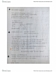# ECON 102 Study Guide - Quiz Guide: Demand Curve, Economic Surplus, Economic Equilibrium

4176 views3 pages
School
Department
Course
Professorapricotsalmon423 and 1 other unlocked13
Verified Note
13 documents

## Document Summary

Price elasticity of demand is a measure of: a. ) Following a price decrease, total revenue will: a. ) Increase if the demand is price inelastic c. ) always increase d. ) always decrease. If the price elasticity of demand for concert tickets is 2. 12, then the demand is _______ and total revenue will ________ if the price of concert tickets increases. a. ) Elastic, increase c. ) elastic, decrease d. ) inelastic, decrease. * since the price elasticity of demand is larger than. This means that when the price increases, demand will be greatly affected, so total revenue will decrease. We would say that apples are a normal good if the. Price elasticity of demand, positive c. ) income elasticity of demand, positive d. ) price elasticity of demand, negative. If two goods have a negative cross-price elasticity of demand between them, we would say that these two goods are: a. )